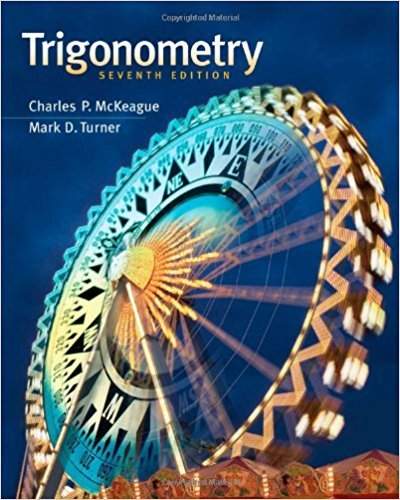×
×

# Solutions for Chapter 1-16: Complex Numbers and Polar Coordinates## Full solutions for Trigonometry | 7th Edition

ISBN: 9781111826857Solutions for Chapter 1-16: Complex Numbers and Polar Coordinates

Solutions for Chapter 1-16
4 5 0 415 Reviews
26
0
##### ISBN: 9781111826857

Since 1 problems in chapter 1-16: Complex Numbers and Polar Coordinates have been answered, more than 25016 students have viewed full step-by-step solutions from this chapter. Chapter 1-16: Complex Numbers and Polar Coordinates includes 1 full step-by-step solutions. This expansive textbook survival guide covers the following chapters and their solutions. Trigonometry was written by and is associated to the ISBN: 9781111826857. This textbook survival guide was created for the textbook: Trigonometry, edition: 7.

Key Math Terms and definitions covered in this textbook
• Basis for V.

Independent vectors VI, ... , v d whose linear combinations give each vector in V as v = CIVI + ... + CdVd. V has many bases, each basis gives unique c's. A vector space has many bases!

• Commuting matrices AB = BA.

If diagonalizable, they share n eigenvectors.

• Cyclic shift

S. Permutation with S21 = 1, S32 = 1, ... , finally SIn = 1. Its eigenvalues are the nth roots e2lrik/n of 1; eigenvectors are columns of the Fourier matrix F.

• Diagonalization

A = S-1 AS. A = eigenvalue matrix and S = eigenvector matrix of A. A must have n independent eigenvectors to make S invertible. All Ak = SA k S-I.

• Eigenvalue A and eigenvector x.

Ax = AX with x#-O so det(A - AI) = o.

• Ellipse (or ellipsoid) x T Ax = 1.

A must be positive definite; the axes of the ellipse are eigenvectors of A, with lengths 1/.JI. (For IIx II = 1 the vectors y = Ax lie on the ellipse IIA-1 yll2 = Y T(AAT)-1 Y = 1 displayed by eigshow; axis lengths ad

• Four Fundamental Subspaces C (A), N (A), C (AT), N (AT).

Use AT for complex A.

• Gram-Schmidt orthogonalization A = QR.

Independent columns in A, orthonormal columns in Q. Each column q j of Q is a combination of the first j columns of A (and conversely, so R is upper triangular). Convention: diag(R) > o.

• Hessenberg matrix H.

Triangular matrix with one extra nonzero adjacent diagonal.

• Hypercube matrix pl.

Row n + 1 counts corners, edges, faces, ... of a cube in Rn.

• Jordan form 1 = M- 1 AM.

If A has s independent eigenvectors, its "generalized" eigenvector matrix M gives 1 = diag(lt, ... , 1s). The block his Akh +Nk where Nk has 1 's on diagonall. Each block has one eigenvalue Ak and one eigenvector.

• Markov matrix M.

All mij > 0 and each column sum is 1. Largest eigenvalue A = 1. If mij > 0, the columns of Mk approach the steady state eigenvector M s = s > O.

• Network.

A directed graph that has constants Cl, ... , Cm associated with the edges.

• Pivot.

The diagonal entry (first nonzero) at the time when a row is used in elimination.

• Saddle point of I(x}, ... ,xn ).

A point where the first derivatives of I are zero and the second derivative matrix (a2 II aXi ax j = Hessian matrix) is indefinite.

• Schur complement S, D - C A -} B.

Appears in block elimination on [~ g ].

• Spanning set.

Combinations of VI, ... ,Vm fill the space. The columns of A span C (A)!

• Symmetric factorizations A = LDLT and A = QAQT.

Signs in A = signs in D.

• Toeplitz matrix.

Constant down each diagonal = time-invariant (shift-invariant) filter.

• Unitary matrix UH = U T = U-I.

Orthonormal columns (complex analog of Q).

×